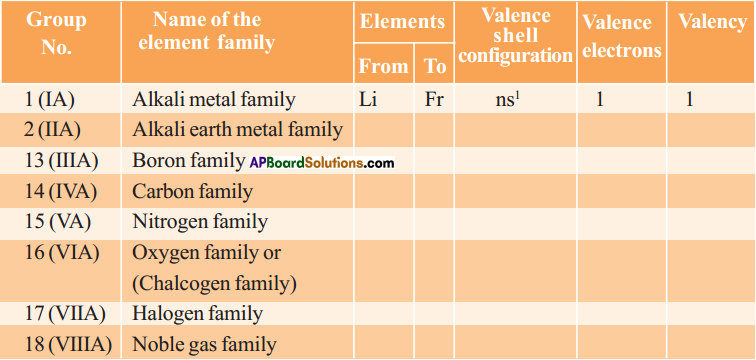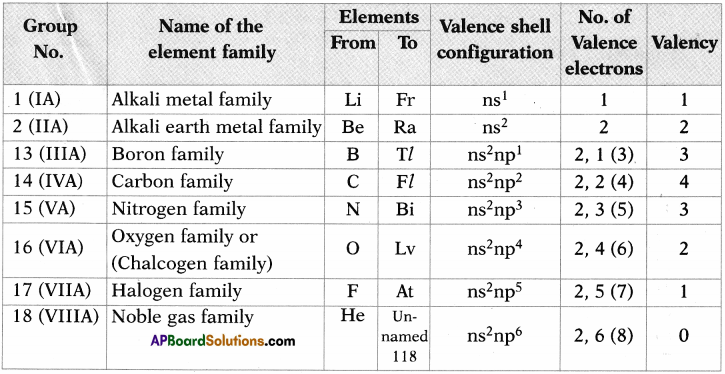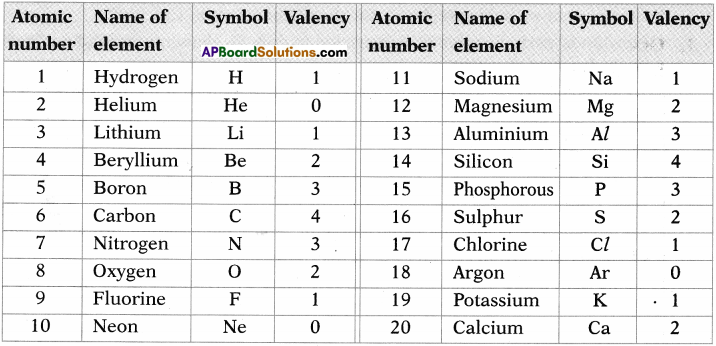AP State Board Syllabus AP SSC 10th Class Chemistry Solutions Chapter 9 Classification of Elements- The Periodic Table Textbook Questions and Answers.

## AP State Syllabus SSC 10th Class Chemistry Solutions 9th Lesson Structure of Atom

### 10th Class Chemistry 9th Lesson Structure of Atom Textbook Questions and Answers

Question 1.
Newlands proposed the law of octaves. Mendeleeff suggested eight groups for elements in his table. How do you explain these observations in terms of modem periodic classification? (AS1)
(OR)
Correlate various tables proposed on classification of elements.

• According to Newlands, every eighth element starting from a given element jsembles in its properties to that of the starting element, when elements are ranged in ascending order of their atomic weights.
• According to Newlands, the properties of fluorine and chlorine are similar and sodium and potassium are similar. Same aspect is given by modern periodic table.
• Mendeleeff divided it into horizontal rows and vertical columns. He called them peribds and groups respectively. Modem periodic table also gives the same.
• According to Mendeleeff, the elements of same group have similar properties. Modern periodic table also proposed the same thing.
• Mendeleeff gave the general formula for first group elements as R,0, and general formula for second group elements as RO. We can find the same thing in modern periodic table.
• The elements of particular group possess same common valency. Same was proposed by modern periodic table.Question 2.
What are the limitations of Mendeleeff’s periodic table? How could the modern periodic table overcome the limitations of Mendeleeff’s table? (AS1)
(OR)
How can the limitations of Mendeleeffs table be overcome with the help of modern periodic table?
Limitations of Mendeleeffs periodic table :
1) Anomalous pair of elements :
Certain elements of highest atomic weights precede those with lower atomic weights.
Eg : Tellurium (atomic weight 127.6) precedes iodine (atomic weight 126.9).

2) Dissimilar elements placed together :
a) Elements with dissimilar properties were placed in same group as sub-group A and sub-group Bt
Eg : Alkali metals like Li, Na, K, etc. of IA group have little resemblance with coinage metals like Cu, Ag, Au of IB group.

b) Cl of VII A group is a non-metal and Mn of VII B group is a metal.

Method of overcoming the limitations of Mendeleeffs periodic table by modern periodic table :
1. In modern periodic table, elements are arranged in the ascending order of their atomic numbers. So this arrangement eliminated the problem of anomalous series.
Eg : Though Tellurium (Te) has more atomic weight than Iodine (I), its atomic number is one unit less compared to Iodine.

2. The elements with similar outer shell (valence shell) electronic configurations in their atoms are in the same column called group in modern periodic table. So the elements have similar properties overcoming the Mendeleeffs second limitation.

Question 3.
Define the modern periodic law. Discuss the construction of the long form of the periodic table. (AS1)
(OR)
What are the salient features of modern periodic table?
Modern periodic law :
‘The physical and chemical properties of elements are the periodic function of the electronic configurations of their atoms”.

Construction of the long form periodic table :

1. Based on the modern periodic law, the modern periodic table is proposed.
2. This periodic table is known as long form of the periodic table.
3. Long form periodic table is the graphical representation of Aufbau principle.
4. The modern periodic table has 18 vertical columns called groups and 7 horizontal rows known as periods.
5. There are 18 groups, represented by using Roman numerals I to VIII, with letters A and B in traditional notation, (or) 1 to 18 by Arabic numerals.
6. There are 7 periods. These periods are represented by Arabic numerals 1 to 7.
7. The number of main shells present in the atom of particular atom decides to which period it belongs.
8. First period consists 2 elements, 2nd and 3rd periods contains 8 elements each, 4th and 5th periods contains 18 elements each, 6 period contains 32 elements and 7th period is incomplete.
9. The elements are classified into s, p, d and f block elements.
10. Inert gases are placed in 18th group.Question 4.
Explain how the elements are classified into s, p, d and f-block elements in the periodic table and give the advantage of this kind of classification. (AS1)
(OR)
How is the periodic table classified based upon the entering of differenciating electron? Explain that classification. What is the advantage of such classification?
1) Depending upon which sub-shell the differentiating electron enters, the elements are classified into s, p, d and f-block elements. They are

1. s – block elements,
2. p – block elements,
3. d – block elements,
4. f – block elements.

2) s – block elements :
i) If the differentiating electron enters in s-sub-shell, then the elements are called s-block elements.
ii) IA (1), IIA (2) group elements belong to this block.

3) p – block elements :
i) If the differentiating electron enters in p-sub-shell, then the elements are called p-block elements.
ii) IIIA(13), IV A (14), V A (15), VIA (16), VIIA (17) belong to p-block.

4) d – block elements :
i) If the differentiating electron enters in d-sub-shell, then the elements are called d – block elements.
ii) I B, II B, III B, IV B, V B, VI B, VII B, VIII B belong to d-block elements.
iii) They are also called transition elements.

5) f – block elements :
i) If the differentiating electrons enter in f-sub-shell, then the elements are called f-block elements.
ii) These are divided into two types
a) Lanthanides (41 elements),
b) Actinides (5f elements).
iii) These are also called as inner transition elements.

1) The systematic grouping of elements into groups made the study simple.
2) Each period begins with the electron entering a new shell and ends with the complete filling of s and p-sub-shells of that shell.

Question 5.
Given below is the electronic configuration of elements A, B, C, D. (AS1)

 A) 1s² 2s² 1. Which are the elements coming within the same period? B) 1s² 2s² 2p6 3s² 2. Which are the elements coming within the same group? C) 1s² 2s² 2p6 3s² 3p³ 3. Which are the noble gas elements? D) 1s² 2s² 2p6 4. To which group and period does the element ‘C’ belong?

According to electronic configuration
A = Be B = Mg C = P D = Ne
1. Which are the elements coming within the same period?
A and D i.e. Be and Ne coming within the same period. [They have same valence shell (n = 2)]

2. Which are the ones coming within the same group?
A and B i.e., Be and Mg coming within the same group. [They have same valence subshell with same valency (2s² and 3s²)]

3. Which are the noble gas elements?
D, i.e. Ne is the noble gas element. [It has valency as ‘O’ and it has ‘8’ electrons in valence shell].

4. To which group and period does the element ‘C’ belong?
Element ‘C’ i.e. ‘P’ belongs to 3rd period and VA group.Question 6.
Write down the characteristics of the elements having atomic number 17. (AS1)
1) Electronic configuration ___________
2) Period number _____________
3) Group number _____________
4) Element family ____________
5) No. of valence electrons ___________
6) Valency _____________
7) Metal or non-metal ____________

1. 1s² 2s² 2p6 3s² 3p5
2. 3
3. VII A or 17
4. Halogen family
5. 7
6. 1
7. Non-metal

Question 7.
a) State the number of valence electrons, the group number and the period number of each element given in the following table : (AS1)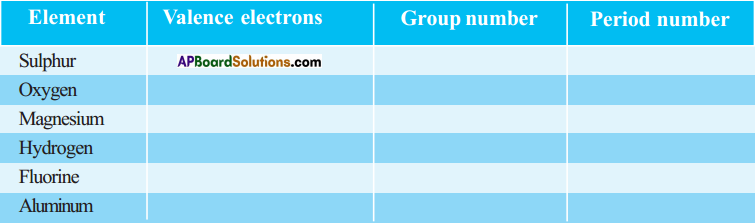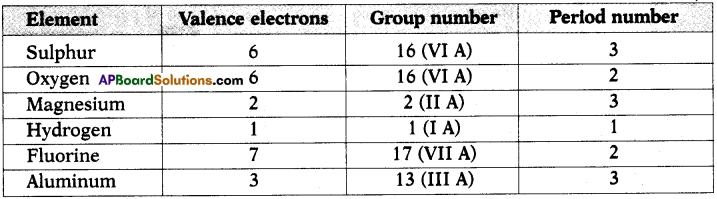b) State whether the following elements belong to a Group (G), Period (P) or neither Group nor Period (N). (AS1)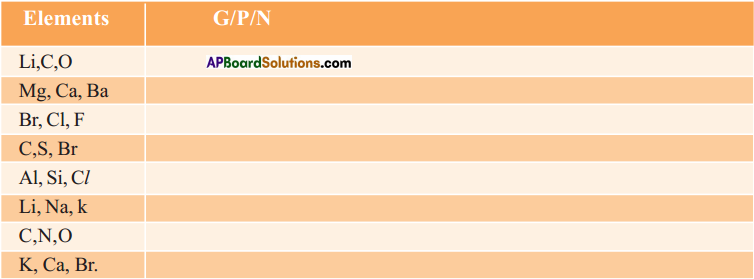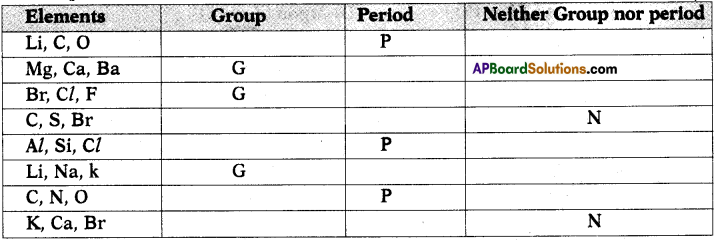Question 8.
Elements in a group generally possess similar properties, but elements along a period have different properties. How do you explain this statement? (AS1)
(OR)
Elements in a group possess similar properties, but elements along a period have different properties. Explain the reason.

• Physical and chemical properties of elements are related to their electronic configurations, particularly the outer shell configurations.
• Therefore, all the elements in a group should have similar chemical properties.
• Similarly, across the table from left to right in any period, elements get an increase in the atomic number by one unit between any two successive elements.
• Therefore, the electronic configuration of valence shell of any two elements in a period is not same. Due to this reason, elements along a period possess different chemical properties.Question 9.
s – block and p – block elements except 18th group elements are sometimes called as ‘Representative elements’ based on their abundant availability in the nature. Is it justified? Why? (AS1)
(OR)
Which elements are called representative elements? Why?

• s, p – block elements are called representative elements because these are the elements which take part in chemical reactions because of incompletely filled outermost shell.
• These elements undergo chemical reactions to acquire the nearest noble gas configuration by losing or gaining or sharing of electrons.
• So they are called representative elements.

Question 10.
Complete the following table using periodic table. (AS1)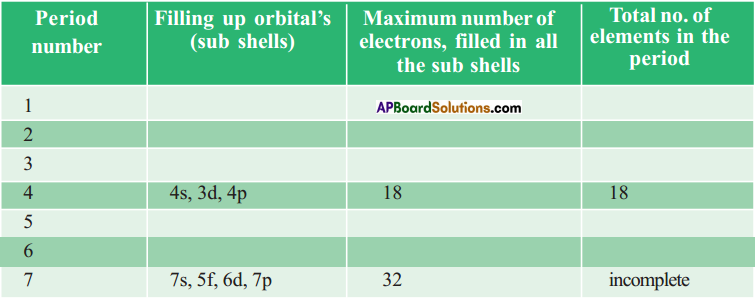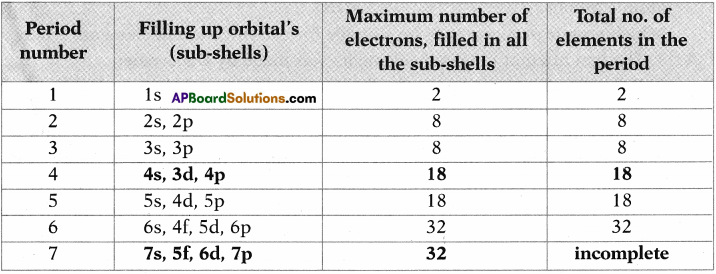Question 11.
Complete the following table using the periodic table. (AS1)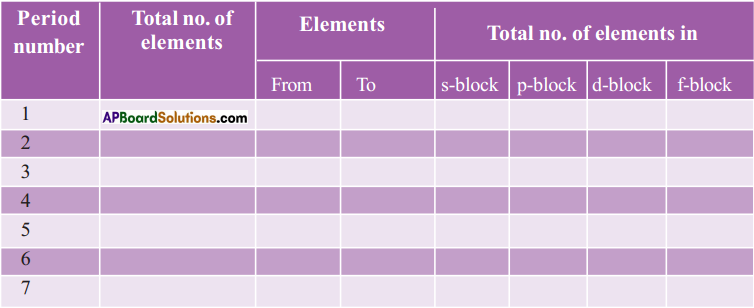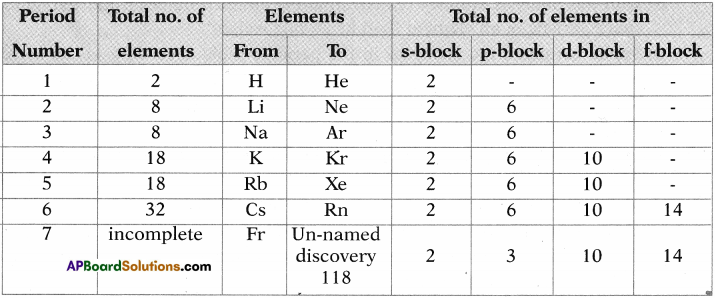Question 12.
The electronic configuration of the elements X, Y, and Z are given below.
a) X = 2
b) Y = 2, 6
c) Z = 2, 8, 2
i) Which element belongs to second period?
Y belongs to second period.

ii) Which element belongs to second group?
Z belongs to second group,

iii) Which element belongs to 18th group?
X belongs to 18th group.Question 13.
Identify the element that has the larger atomic radius in each pair of the following and mark it with a symbol (✓). (AS1)
(i) Mg or Ca
(ii) Li or Cs
(iii) N or P
(iv) B or Al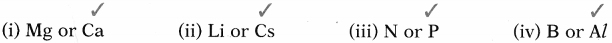Question 14.
Identify the element that has the lower ionization energy in each pair of the, following and mark it with a symbol (✓). (AS1)
(i) Mg or Na (ii) Li or O (iii) Br or F (iv) K or Br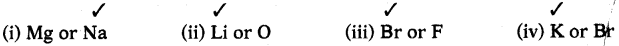Question 15.
In period 2, element X is to the right of element Y. Then, find which ofitheydements have : (AS1)
i) Low nuclear charge
Y has low nuclear charge.

ii) Low atomic size
X has lower atomic size,

iii) High ionization energy
X has higher ionization energy.

iv) High electronegativity
Xhas high electronega^vity.

v) More metallic,character
Y has more metallic character.

Question 16.
How does metallic character change when we move
i) Down a group?
ii) Across a period?
i) Down a group :
When we move from top to bottom in a group, the metallic character increases.

ii) Across a period:
When we move left to right in a period, the metallic character decreases.Question 17.
Why was the basis of classification of elements changed from the atomic mass to the atomic number? (AS1)
(OR)
Which atomic property is more suitable for classification of elements? Why?

• The first attempt to classify elements was made by Dobereiner.
• Dobereiner’s attempt gave a clue that atomic masses could be correlated with properties of elements:
• Newlands’ law of octaves also followed the same basis for classification but this law is not valid for the elements that had atomic masses higher than calcium.
• Mendeleeff’s classification also based on the atomic masses of elements, but it lead to some limitations like Anomalous pair of elements and Dissimilar elements placed together.
• Moseley by analyzing the X-ray patterns of different elements was able to calculate the number of positive charges in the atoms of respective elements.
• With this analysis, Moseley realized that the atomic number is more fundamental
characteristic of an element than its atomic weight. ,
• So, he arranged the elements in the periodic table according to the increasing order of their atomic number.
• This arrangement eliminated the problem of anomalous series and dissimilar elements placed together in Mendeleeff’s classification.

Question 18.
What is a periodic property? How do the following properties change in a group and period? Explain. (AS1)
b) Ionization energy
c) Electron affinity
d) Electronegativity
II. Explain the ionization energy order in the following sets of elements: (AS1)
a) Na, Al, Cl
b) Li, Be, B
c) C, N, O
d) F, Ne, Na
e) Be, Mg, Ca
Periodic property:
The property in which there shall be a regular gradation is called periodic property.

Period :
Atomic radius of elements decreases across a period from left to right because the nuclear charge increases due to increase in atomic number.

Group :
Atomic radius increases from top to bottom in a group due to addition of new shell.

b) Ionization energy:
Period :
When we move from left to right it does not follow a regular trend but generally increases due to increase in atomic number.

Group :
In a group from top to bottom, the ionization energy decreases due to increase in atomic size. –

c) Electron affinity:
Period :
Electron affinity values increase from left to right in a period.

Group :
Electron affinity values decrease from top to bottom in a group.

d) Electronegativity :
Period :
Electronegativity increases from left to right in a period.

Group :
Electronegativity decreases from top to bottom in a group.

II. Ionization energy order :
a) Na, Al, Cl
b) Li, Be, B
c) C, N, O
d) F, Ne, Na
e) Be, Mg, Ca
a) In a period ionisation energy increases so the order is Na < kl < Cl.
b) Beryllium has stable configuration 1s² 2s². So it has more ionisation energy. So the order is Li < B < Be.
c) Nitrogen has half-filled p-orbitals. So it has greater ionisation energy. So the order is C < O < N.
d) Ne is inert gas right to F. Whereas Na is a metal ion in third period. So, the order is Na < F < Ne. e) In a group ionisation energy decreases. So the order is Be > Mg > Ca.Question 19.
Name two elements that you would expect to have chemical properties similar to Mg. What is the basis for your choice? (AS2)

• The two elements which have chemical properties similar to Magnesium are Beryllium and Calcium.
• The basis for my expectation is that they belong to same group as we know elements belonging to same group have similar properties.

Question 20.
On the basis of atomic numbers predict to which block the elements with atomic number 9, 37, 46 and 64 belong to? (AS2)

1. The element with atomic number 9 belongs to p-block.
2. The element with atomic number 37 belongs to s-block.
3. The element with atomic number 46 belongs to d-block.
4. The element with atomic number 64 belongs to f-block.

Question 21.
Using the periodic table, predict the formula of compound formed between and element X of group 13 and another element Y of group 16. (AS2)
The valency of 13th group elements is 3.
The valency of 16th group elements is 2.
The formula of compound is X2Y3.

Question 22.
An element X belongs to 3rd period and group 2 of the periodic table. State (AS2)
a) The no. of valence electrons
b) The valency.
c) Whether it is metal or a non-metal.
a) The number of valence electrons are 2.
b) The valency of element is +2.
c) It is a metal.Question 23.
An element has atomic number 19. Where would you expect this element in the periodic table and why? (AS2)
The clement with atomic number 19 is in 4th period and first group of the periodic table.
Reason :

1. Electronic configuration : 1s² 2s² 2p6 3s² 3p6 4s or [Ar]4s¹
2. The differentiating electron enters into 4th shell. Hence it belongs to 4th period.
3. The differentiating electron is in ‘s’ orbital. So it belongs to ‘s’ block.
4. The outermost orbital has only one electron. Hence it belongs to first group.

Question 24.
Aluminium does not react with water at room temperature but reacts with both dil. HCl and NaOH solutions. Verify these statements experimentally. Write your observations with chemical equations. From these observations, can we conclude that Al is a metalloid? (AS3)

• Aluminium reacts with dil. HCl and releases hydrogen gas with formation of Aluminium chloride.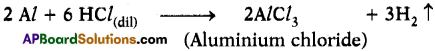• Aluminium reacts with NaOH solution and releases hydrogen gas.
•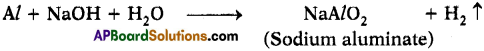• The above two reactions says that Aluminium is amphoteric.
• Aluminium does not react with water at room temperature.
• This concludes that the properties of Aluminium are in between a metal and non¬metal. So it behaves like a metalloid.

Question 25.
Collect the information about reactivity of VIIIA group elements (noble gases) from internet or from your school library and prepare a report on their special character when compared to other elements of periodic table. (AS4)
Reactivity of Noble gases :

• The noble gases show extremely low chemical reactivity.
• He and Ne do not form chemical compounds.
• Xenon, krypton and argon show only minor reactivity.
• The reactivity order follows like this : Ne < He < Ar < Kr < Xe < Rn.
• Xenon can form compounds like XeF2, XeF4 and XeF6, etc.

Reasons for low reactivity :

• The extremely low reactivity of noble gases is due to stable electronic configuration.
• But as we move from top to bottom the reactivity increases. So xenon can form some compounds with high electronegative elements.Question 26.
Collect information regarding metallic character of elements of IA group and prepare report to support the idea of metallic character increases in a group as we move from top ro bottom. (AS4)
Metallic character of IA group elements :

1. Alkali metals exhibit many of the physical properties common to metals but their densities are lower than those of other metals.
2. Alkali metals have one electron in their outer shell which is loosely bound.
3. They have largest atomic radii of the elements in their respective periods.
4. The lower ionization energies result in their metallic properties and high reactivities.
5. An alkali metal can easily lose its valence electron to form positive ion.
6. So they have greater metallic character.
7. The metallic character increases as we move from top to bottom in group due to addition of another shell, it is easy to lose electron.

Question 27.
How do you appreciate the role of electronic configuration of the atoms of elements in periodic classification? (AS6)
(OR)
How does electronic configuration help in the classification of elements in modern periodic table?
The quantity is electronic configuration.

1. Modern periodic table is based on electronic configuration. So elements are arranged in ascending order of their atomic numbers.
2. The chemical properties of elements depend on valence electrons. The elements in same group have same number of valence electrons. So the elements belonging to same group have similar properties.
3. So the construction of modern periodic table mainly depends on electronic configuration.
4. Thus electronic configuration plays a major role in the preparation of modern periodic table. So its role is thoroughly appreciated.

Question 28.
Without knowing the electronic configurations of the atoms of elements Mendeleeff still could arrange the elements nearly close to the arrangements in the Modern periodic table. How can you appreciate this? (AS6)

• Mendeleeff took consideration about chemical properties while arranging the elements. So the arrangement of elements is close to arrangement of elements in Modern periodic table.
• For this, he violated his periodic law.
• He left some gaps for elements, later those elements are discovered.
• So the efforts of Mendeleeff should be thoroughly appreciated.

Question 29.
Comment on the position of hydrogen in periodic table. (AS7)

• Hydrogen is the element which has easier atomic structure than any other element.
• Electron configuration of hydrogen is Is1. It has one proton in its is nucleus and one electron in its is orbital.
• Hydrogen combines with halogens, oxygen and sulphur to form compounds having similar formulae just like alkali metals.
• Similarly, just like halogens, hydrogen also exists as diatomic molecule and combine with metals and non-metals to form covalent compounds.
• As alkali metals hydrogen can lose one electron and accept one electron as halogens.
• So in periodic table, its place may be in IA or VIIA group.
• But based on electronic configuration of hydrogen, it is placed in IA group.Question 30.
How do the positions of elements in the periodic table help you to predict its chemical properties? Explain with an example. (As7)
1) The physical and chemical properties of atoms of the elements depend on their electronic configuration, particularly the outer shell configurations.

2) Elements are placed in the periodic table according to the increasing order of their electronic configuration.

3) The elements in a group possess similar electronic configurations. Therefore all the elements in a group should have similar chemical properties.
Ex : Consider K

• It is the element in 4th period 1st group.
• Electron configuration : 1s² 2s² 2p6 3s² 3p6 4s¹.
• Differentiating electron enters into s-orbital. Hence it belongs to s-block.
• It is on the left side of the periodic table. Hence it is a metal.
• It is ready to lose one electron to get octet configuration. Hence its reactivity is more.
• It is Alkali metal.
• All alkali metals react with both acids and bases and releases H2 gas.

Fill In The Blanks

1. Lithium, ……………… and potassium constitute a Dobereiner’s triad.
2. ……………… was the basis of the classifications proposed by Dobereiner, Newlands, and Mendeleeff.
3. Noble gases belong to ……………… group of periodic table.
4. The incomplete period of the modern periodic table is
5. The element at the bottom of a group would be expected to show …………….. metallic character than the element at the top

1. Sodium
2. Atomic weight
3. VIIIA or 18 group
4. 7<sup>th</sup>
5. higher

Multiple Choice Questions

1. Number of elements present in period – 2 of the long form of periodic table …………
A) 2
B) 8
C) 18
D) 32
B) 8

2. Nitrogen (Z = 7) is the element of group V of the periodic table. Which of the following is the atomic number of the next element in the group?
A) 9
B) 14
C) 15
D) 17
C) 153. Electronic configuration of an atom is 2, 8, 7. To which of the following elements would it be chemically similar?
A) Nitrogen (Z = 7)
B) Fluorine (Z = 9)
C) Phosphorous (Z – 15)
D) Argon (Z = 18)
B) Fluorine (Z = 9)

4. Which of the following is the most active metal?
A) lithium
B) sodium
C) potassium
D) rubidium
D) rubidium

### 10th Class Chemistry 9th Lesson Classification of Elements-The Periodic Table InText Questions and Answers

10th Class Chemistry Textbook Page No. 129

Question 1.
Can you establish the same relationship with the set of elements given in the remaining rows?
Yes, we can establish the approximately same relationship between other elements given in the table.

Question 2.
Find average atomic weights of the first and third elements in each row and compare it with the atomic weight of the middle element. What do you observe?
The atomic weight of middle element is arithmetic mean coverage of first and third elements.

10th Class Chemistry Textbook Page No. 135

Question 3.
What is atomic number?
The number of positive charges (protons) in the atom of element is the atomic number of element.

10th Class Chemistry Textbook Page No. 142

Question 4.
How does the valency vary in a period on going from left to right?
It does not follow a regular trend when we move from left to right in a period. First, it increases and then decreases and finally ‘O’ for inert gases.Question 5.
How does the valency vary on going down a group?
The valency is constant when we move from top to bottom in a group because the number of valence electrons are same for same group elements.

10th Class Chemistry Textbook Page No. 144

Question 6.
Do the atom of an element and its ion have same size?
No, the positive ion has smaller size than neutral atom whereas negative ion has greater size than neutral atom.

Question 7.
Which one between Na and Na+ would have more size? Why?

• The atomic number of Sodium is 1 and it has 11 protons and 11 electrons with outer electron as 3s¹ whereas Na+ ion has 11 protons but only 10 electrons.
• The 3s shell of Na+ has no electron in it.
• So the outer shell configuration is 2s²2p6.
• As proton number is more than electrons, the nucleus of Na+ ion attracts outer shell electrons with strong nuclear force.
• As a result the Na+ ion shrinks in size.
• Therefore, the size of Na+ ion is less than Na atom.Question 8.
Which one between Cl and Cl would have more size? Why?

• The electronic configuration of chlorine (Cl) atom is 1s2 2s² 2p6 3s² 3p5 and the electronic configuration of chloride (Cl) ion is 1s² 2s² 2p6 3s² 3p6.
• Both chlorine and chloride ions have 17 protons each but there are 17 electrons in chlorine atom, whereas 18 electrons in chloride ion.
• Therefore, the nuclear attraction is less in Cl ion when compared with chlorine atom.
• Therefore the size of the chlorine (Cl) atom is less size than chloride of Cl ion.

Question 9.
Which one in each of the following pairs is larger in size? Why?
a) Na, Al
b) Na, Mg+2
c) S2-, Cl
d) Fe2+, Fe3+
e) C4-, F.
a) Na has larger size because Sodium and Aluminium are third period elements in which Na is left to Al. As we move from left to right in a period atomic size decreases.

b) Mg2+ has smaller size because Mg2+ has 10 electrons and 12 protons whereas Na has 11 electrons and 11 protons. So the distance between nucleus and outermost orbital is less in Mg2+ due to greater nuclear attraction.

c) S2- has, larger size because S2- has 18 electrons and 16 protons and Cl has 18 electrons and 17 protons. So nuclear attraction over outermost orbital is more in Cl when compared with S2-. So S2- has larger size.

d) In Fe2+ it has 26 protons and 24 electrons whereas for Fe3+ it has 26 protons and 23 electrons. So nuclear attraction over outermost orbital is more in Fe3+. So Fe3+ has smaller size (or) Fe2+ has larger size.

e) C4- has 6 protons and 10 electrons whereas F has 9 protons and 10 electrons. So nuclear attraction is less in C4-. So size of C4- is more than F.

10th Class Chemistry Textbook Page No. 129

Question 10.
What relation about elements did Dobereiner want to establish?
Dobereiner wanted to give a relationship between the properties of elements and their atomic weights.

Question 11.
The densities of calcium (Ca) and barium (Ba) are 1.55 and 3.51 gem-3 respectively. Based on Dobereiner’s law of triads can you give the approximate density of strontium (Sr)?
Molecular weight is directly proportional to density.

So density of strontium is mean of calcium and barium according to Dobereiner.
∴ Density of strontium = $$\frac{1.55+3.51}{2}$$ = 2.53.

10th Class Chemistry Textbook Page No. 130

Question 12.
Do you know why Newlands proposed the law of octaves? Explain your answer in terms of the modern structure of the atom.

• John Newlands found that when elements were arranged in the ascending order of their atomic weights, they appeared to fall into seven groups.
• Each group contained elements with similar properties.
• If we start with hydrogen and move down, the next eighth element is fluorine, and then next eighth element is chlorine and the properties of these elements are similar.
• Similarly, if we start from Lithium their eighth element is Sodium and next eighth element is potassium. These show similar properties.Question 13.
Do you think that Newlands’ law of octaves is correct? Justify.
No, there are some limitations of Newlands’ model:

• There are instances of two elements fitted into the same slot. Eg : Cobalt and Nickel.
• Certain elements, totally dissimilar in their properties, were fitted into the same group.
• Law of octaves holds good only for the elements up to Calcium.
• Newlands’ periodic table was restricted to only 56 elements and did not leave any room for new elements.
• Newlands had taken consideration about active pattern sometimes without caring the similarities.

10th Class Chemistry Textbook Page No. 134

Question 14.
Why did Mendeleeff have to leave certain blank spaces in his periodic table? What is your explanation for this?
1) Mendeleeff predicted that some elements which have similar properties with the elements in a group are missing at that time.
2) So he kept some blanks in the periodic table by writing ’eka’ to the name of the element immediately above the empty space.
3) Later these elements are discovered and they are fitted into those empty spaces.

Question 15.

• Mendeleeff predicted that after aluminium there was another element namely eka- aluminium (Ea) and after silicon, there was another element namely eka-silicon (Es).
• He also gave the formulae of those oxides as Ea203 and Es02.
• Later those elements are discovered namely gallium and germanium and Ea2O3 and EsO2 as Ga2O3 and GeO2.

10th Class Chemistry Textbook Page No. 135

Question 16.
All alkali metals are solids but hydrogen is a gas with diatomic molecules. Do you justify the inclusion of hydrogen in first group with alkali metals?
No, hydrogen shows the properties of both alkali metals and halogens. Still the position of hydrogen has some questions. So it was kept just above alkali metals in first group.

10th Class Chemistry Textbook Page No. 141

Question 17.
Why are lanthanoids and actinoids placed separately at the bottom of the periodic table?
The properties of these elements do not coincide with other elements because the valence electron enters 4f and 5f orbitals respectively. So they are placed separately at the bottom of the periodic table.

Question 18.
If lanthanoids and actinoids are inserted within the table, imagine how the table would be?
It looks very big in size, and it is difficult to identify, as these elements have similar properties.

10th Class Chemistry Textbook Page No. 145

Question 19.
Second ionization energy of an element is higher than its first ionization energy. Why?

• The energy required to remove an electron from unipositive ion is called second ionisation energy.
• It is difficult to remove an electron from unipositive ion when compared with neutral atom due to an increase in nuclear attraction.
• So always second ionisation energy is higher than first ionisation energy.

10th Class Chemistry Textbook Page No. 146

Question 20.
The calculated electron gain enthalpy values for alkaline earth metals and noble gases are positive. How can you explain this?

• Generally alkaline earth metals having one, two or three valence electrons prefer to lose electrons in order to get inert gas configuration. So it is difficult to add electron to alkaline earth metals. So they have positive electron gain enthalpy values.
• Noble gases are stable. So they do not prefer to take electrons. So they have positive electron gain enthalpy.Question 21.
The second period element, for example, ‘F’ has less electron gain enthalpy than the third period element of the same group for example ‘Cl’. Why?

• Electron gain enthalpy values decrease in a group as we go down and increase from left to right along a period.
• But the size of Fluorine is small compared chlorine.
• So it is difficult to add electron to fluorine.
• So fluorine has less electron gain enthalpy.

10th Class Chemistry 9th Lesson Classification of Elements- The Periodic Table Activities

Activity – 1

Question 1.
Observe the following table. Establish the relationship of other elements given in the table.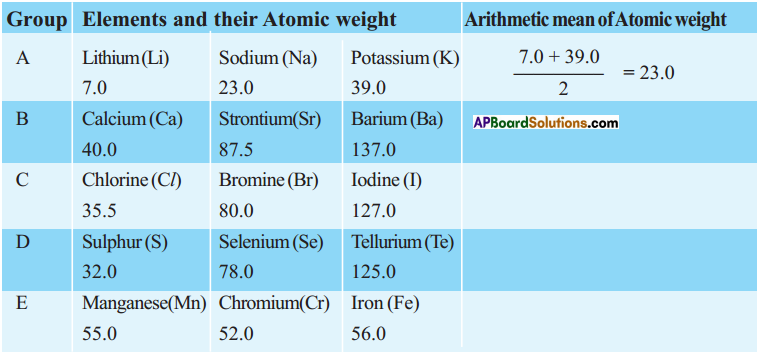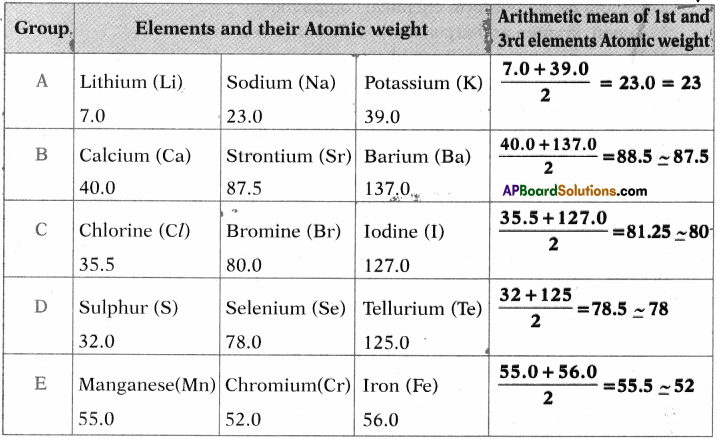Activity – 2

Question 2.
Some main group elements of s-block and p-block have family names as given in the following table.
Observe the long form of a periodic table and complete the table with proper information.